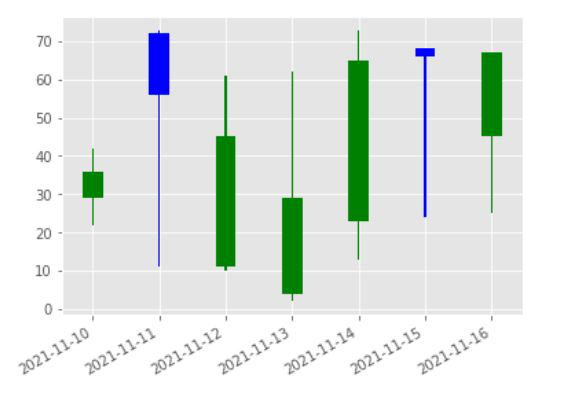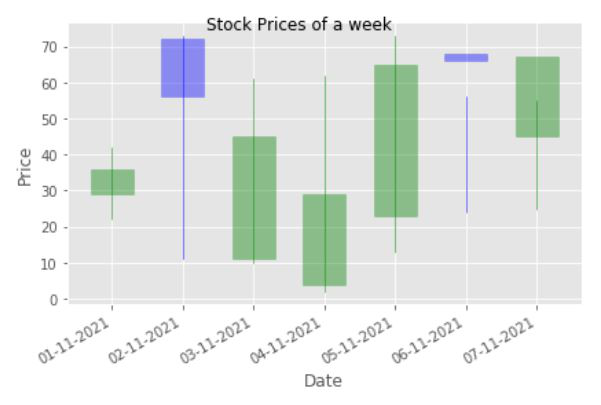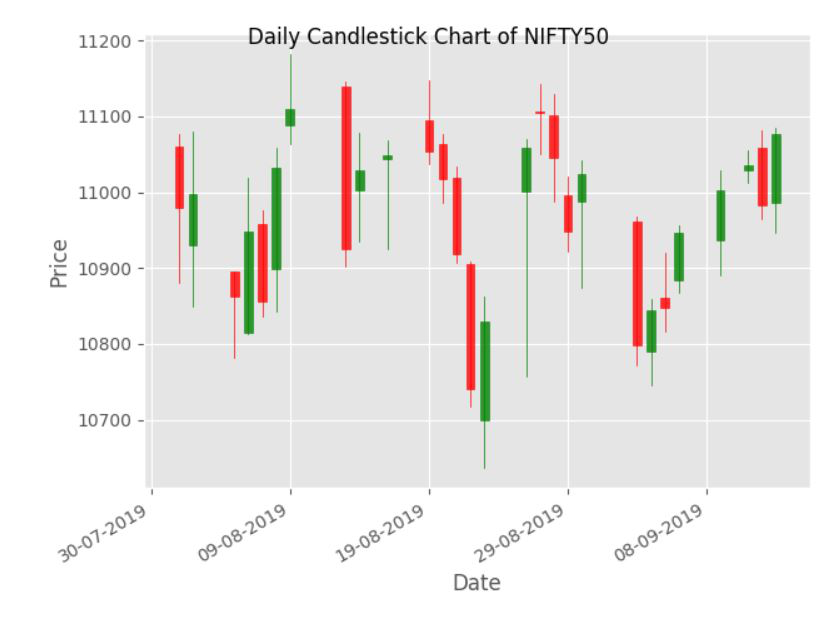# How to Create a Candlestick Chart in Matplotlib?

• Last Updated : 28 Nov, 2021

A candlestick chart, often known as a Japanese candlestick chart, is a financial chart that shows the price movement of stocks, derivatives, and other financial instruments in real-time, there are simply four essential components that must be examined. The open, high, low, and close are the four key elements, the candlestick chart has been used. It’s one of the world’s oldest charts.

The syntax of making a candlestick chart is as follows. Here we have used plt.bar method to build the candlestick chart.

Syntax:

plt.bar(up.index,up.close-up.open,bottom=up.open,color)

where,

• “up” dataframe will store the stock_prices when the closing stock price is greater than or equal to the opening stock prices

plt.bar(down.index,down.close-down.open,bottom=down.open,color)

where ,

• “down” dataframe will store the stock_prices when the closing stock price is lesser than the opening stock prices

Example 1: Python program to plot 4 columns with up and down sticks

## Python3

 `import` `pandas as pd``import` `matplotlib.pyplot as plt`` ` `# DataFrame to represent opening , closing, high ``# and low prices of a stock for a week``stock_prices ``=` `pd.DataFrame({``'open'``: [``36``, ``56``, ``45``, ``29``, ``65``, ``66``, ``67``],``                             ``'close'``: [``29``, ``72``, ``11``, ``4``, ``23``, ``68``, ``45``],``                             ``'high'``: [``42``, ``73``, ``61``, ``62``, ``73``, ``56``, ``55``],``                             ``'low'``: [``22``, ``11``, ``10``, ``2``, ``13``, ``24``, ``25``]},``                            ``index``=``pd.date_range(``                              ``"2021-11-10"``, periods``=``7``, freq``=``"d"``))`` ` ` ` `plt.figure()`` ` `# "up" dataframe will store the stock_prices ``# when the closing stock price is greater``# than or equal to the opening stock prices``up ``=` `stock_prices[stock_prices.close >``=` `stock_prices.``open``]`` ` `# "down" dataframe will store the stock_prices``# when the closing stock price is``# lesser than the opening stock prices``down ``=` `stock_prices[stock_prices.close < stock_prices.``open``]`` ` `# When the stock prices have decreased, then it``# will be represented by blue color candlestick``col1 ``=` `'blue'`` ` `# When the stock prices have increased, then it ``# will be represented by green color candlestick``col2 ``=` `'green'`` ` `# Setting width of candlestick elements``width ``=` `.``3``width2 ``=` `.``03`` ` `# Plotting up prices of the stock``plt.bar(up.index, up.close``-``up.``open``, width, bottom``=``up.``open``, color``=``col1)``plt.bar(up.index, up.high``-``up.close, width2, bottom``=``up.close, color``=``col1)``plt.bar(up.index, up.low``-``up.``open``, width2, bottom``=``up.``open``, color``=``col1)`` ` `# Plotting down prices of the stock``plt.bar(down.index, down.close``-``down.``open``, width, bottom``=``down.``open``, color``=``col2)``plt.bar(down.index, down.high``-``down.``open``, width2, bottom``=``down.``open``, color``=``col2)``plt.bar(down.index, down.low``-``down.close, width2, bottom``=``down.close, color``=``col2)`` ` `# rotating the x-axis tick labels at 30degree ``# towards right``plt.xticks(rotation``=``30``, ha``=``'right'``)`` ` `# displaying candlestick chart of stock data ``# of a week``plt.show()`

Output:We can also make a candlestick chart by using the mpl_finance module. To use mpl_finance we need to install it first, which can be done by using the code. We have to install mpl_finance.

`pip install mpl_finance`

Syntax:

candlestick_ohlc(ax, ohlc.values, width, colorup, colordown)

where

• ac is the axis
• values are the input values
• width is the width of each candle stick
• colorup is the color for up sticks
• colordown is the color for down sticks

Example 2: Here, we define a dataset of stock prices that contains 5 parameters i.e open, close, high, low, and index (i.e date) and after that, we used pandas.to DateTime to convert the date, and then pandas.astype to convert all of the data to float ().

## Python3

 `# Importing all the required libraries`` ` `import` `matplotlib.pyplot as plt``from` `mpl_finance ``import` `candlestick_ohlc``import` `pandas as pd``import` `matplotlib.dates as mpl_dates``import` `numpy as np``import` `datetime`` ` ` ` `# Defining a dataframe showing stock prices ``# of a week``stock_prices ``=` `pd.DataFrame({``'date'``: np.array([datetime.datetime(``2021``, ``11``, i``+``1``)``                                               ``for` `i ``in` `range``(``7``)]),``                             ``'open'``: [``36``, ``56``, ``45``, ``29``, ``65``, ``66``, ``67``],``                             ``'close'``: [``29``, ``72``, ``11``, ``4``, ``23``, ``68``, ``45``],``                             ``'high'``: [``42``, ``73``, ``61``, ``62``, ``73``, ``56``, ``55``],``                             ``'low'``: [``22``, ``11``, ``10``, ``2``, ``13``, ``24``, ``25``]})`` ` `ohlc ``=` `stock_prices.loc[:, [``'date'``, ``'open'``, ``'high'``, ``'low'``, ``'close'``]]``ohlc[``'date'``] ``=` `pd.to_datetime(ohlc[``'date'``])``ohlc[``'date'``] ``=` `ohlc[``'date'``].``apply``(mpl_dates.date2num)``ohlc ``=` `ohlc.astype(``float``)`` ` `# Creating Subplots``fig, ax ``=` `plt.subplots()`` ` `candlestick_ohlc(ax, ohlc.values, width``=``0.6``, colorup``=``'blue'``,``                 ``colordown``=``'green'``, alpha``=``0.4``)`` ` `# Setting labels & titles``ax.set_xlabel(``'Date'``)``ax.set_ylabel(``'Price'``)``fig.suptitle(``'Stock Prices of a week'``)`` ` `# Formatting Date``date_format ``=` `mpl_dates.DateFormatter(``'%d-%m-%Y'``)``ax.xaxis.set_major_formatter(date_format)``fig.autofmt_xdate()`` ` `fig.tight_layout()`` ` `plt.show()`

Output:We can also use a dataframe rather than defining it on our own. The dataset can be downloaded by clicking here.

Example 3:

## Python3

 `import` `matplotlib.pyplot as plt``from` `mpl_finance ``import` `candlestick_ohlc``import` `pandas as pd``import` `matplotlib.dates as mpl_dates``import` `numpy as np``import` `datetime`` ` ` ` `# Extracting Data for plotting``data ``=` `pd.read_csv(``"C:/Users/aparn/Desktop/data.csv"``)``ohlc ``=` `data.loc[:, [``'Date'``, ``'Open'``, ``'High'``, ``'Low'``, ``'Close'``]]`` ` `# Converting date into datetime format``ohlc[``'Date'``] ``=` `pd.to_datetime(ohlc[``'Date'``])``ohlc[``'Date'``] ``=` `ohlc[``'Date'``].``apply``(mpl_dates.date2num)``ohlc ``=` `ohlc.astype(``float``)`` ` `# Creating Subplots``fig, ax ``=` `plt.subplots()`` ` `candlestick_ohlc(ax, ohlc.values, width``=``0.6``,``                 ``colorup``=``'green'``, colordown``=``'red'``, alpha``=``0.8``)`` ` `# Setting labels & titles``ax.set_xlabel(``'Date'``)``ax.set_ylabel(``'Price'``)``fig.suptitle(``'Daily Candlestick Chart of NIFTY50'``)`` ` `# Formatting Date``date_format ``=` `mpl_dates.DateFormatter(``'%d-%m-%Y'``)``ax.xaxis.set_major_formatter(date_format)``fig.autofmt_xdate()`` ` `fig.tight_layout()`` ` `plt.show()`

Output:My Personal Notes arrow_drop_up